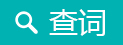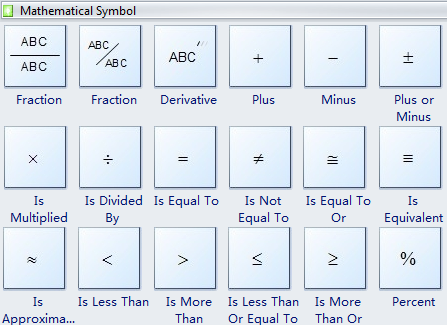# 英文中各种符号标点都怎么说？

#### 中国日报网双语新闻微信 2016-02-24 13:40

2x(-4)=-8Mathematical symbols

01

+: plus 加号；正号

“1+1”读作：one plus one

02

－: minus; negative 减号；负号

“2-1”读作：two minus one

“-3”读作：minus three或negative three

“2-(-3)”读作：two minus negative three

03

±: plus-minus sign 正负号

“±1” 读作：plus or minus one

04

×: multiplied by ; times 乘号

“2×3” 读作：two multiplied by three 或 two times three

05

÷: divided by 除号

“4÷2”读作：four divided by two

06

=: equals; is equal to 等于号

“1+1=2”读作：One plus one equals two.

07

≠: is not equal to 不等于号

“a≠b”读作：a is not equal to b

08

≌: congruent to 全等（两个图形形状相同、大小相等）

“△ABC≌△DEF”读作：Triangle ABC is congruent to Triangle DEF.

09

≈: approximately equal to 约等于号

“3×3≈10” 读作：Three multiplied by three is approximately equal to ten.

10

<: is less than 小于号

“1<2”读作 One is less than two.

11

>: is greater than 大于号

“2>1”读作：Two is greater than one.

12

≮: is not less than 不小于号

“3≮2”读作：Three is not less than two.

13

≯: is not greater than 不大于号

“2≯4” 读作：Two is not greater than four.

14

≤: is less than or equal to 小于或等于号

“2≤3”读作：Two is less than or equal to three.

15

≥: is greater than or equal to 大于或等于号

“5≥4”读作：Five is greater than or equal to four.

16

%: percent 百分之……

“10%”读作：ten percent

17

‰ : per mill ; per thousand 千分之……

“10‰”读作：ten per thousand

18

∞: infinity 无限大号

“－∞” 读作：negative infinity

19

∝: varies as 与...成比例

“X∝Y”读作：X varies as Y

20

√￣: square root 平方根

“√￣16=4”读作：The square root of sixteen is four.

21

3√￣: cube root 立方根

“3√￣27=3”读作：The cube root of 27 is 3.

22

∵: since; because 因为

“∵1+1=2”读作：because one plus one equals two

23

∴: hence; therefore 所以

“∴1<2”读作：hence one is less than two

24

∠: angle 角

“∠A”读作：angle A

“∠A +∠B+∠C=180°”

25

⌒: semicircle 半圆

“⌒AB”读作：semicircle AB

26

⊙: circle 圆

“⊙A”读作：circle A

27

○: circumference 圆周

“○=π×d”读作：Pi multiplied by d equals circumference.

28

π: pi constant 圆周率

“π×d”读作：pi multiplied by d

29

△: triangle 三角形

“△ABC”读作：triangle ABC

What is the area of triangle ABC? 求三角形ABC的面积。

30

⊥: perpendicular to 垂直于

“A⊥B”读作：A is perpendicular to B.

31

//: parallel to 平行于

Line AB is parallel to line CD.

32

∪: union of 并，合集

“A∪B”读作：the union of A and B

33

∩: intersection of 交，通集

“A∩B”读作：The intersection of A and B

34

∫: the integral of ……的积分

“∫x”读作：the integral of x

35

∑: summation of 总和

“∑x”读作：the summation of x

36

equation 方程式、等式

“y = kx” 读作：

Y varies as x.

K is "the constant of variation.

y与x成正比（y是x的正比函数），k是常数。

“y = k/x”读作：

Y varies inversely as x.

y与x成反比例（y是x的反比例函数）。

### 你可能还感兴趣的文章### 中国日报网双语新闻

#### 扫描左侧二维码######Copyright by chinadaily.com.cn. All rights reserved. None of this material may be used for any commercial or public use. Reproduction in whole or in part without permission is prohibited. 版权声明：本网站所刊登的中国日报网英语点津内容，版权属中国日报网所有，未经协议授权，禁止下载使用。 欢迎愿意与本网站合作的单位或个人与我们联系。